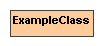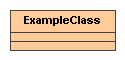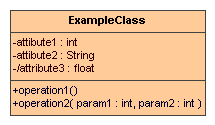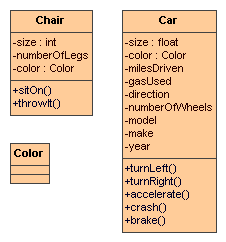## UML notation

UML stands for Unified Modelling Language.

UML consists of different types of diagrams.

We will be looking into class diagrams.

Two types of information:

• Relations between classes (later)

There are two different notation for classes. The shortened notation and the full notation.

Figure 1.1. UML short class notationA class is denoted by a rectangle. The name of the class is written in bold font. The color is just for illustration.

Figure 1.2. UML long class notationIn the long notation the UML class gets divided vertically into 3 compartments:

• the top compartment contains the class name, still in bold

• the second compartment contains the attributes

• the third compartment contains the operations

Compartmets (except for the class name) can be shown as empty, even if there are attributes / operations present, however, all compartments need to be shown.

Figure 1.3. UML long class notation with some attributes and operationsAttributes are shown as follows:

• First the visibility modifier (explanation later) (optional)

• then optional modifiers (explanation later)

• then the name of the attribute (remember: lower case!)

• a colon ( : ) and the type of the attribute (optional)

Operations are shown as follows:

• First the visibility modifier (explanation later)

• then the operation's name

• in parenthesis the list of parameters

• a colon ( : ) and the type of the return value (optional)

Example: Draw class diagrams for the classes, operations, and attributes from the list earlier.

Example 1.2. Example Class DiagramsBook: Chapter 3.1 - 3.3